Mary Ellen WeberEL61/Sun conjunction

>>Mary Ellen Weber, PhD, (born August 24, 1962) is a former NASA astronaut.
Biography
Weber was born in Cleveland, Ohio and raised in Bedford Heights, Ohio. She graduated from Bedford High School in 1980; received a Bachelor of Science degree in chemical engineering (with honors) from Purdue University, where she was a member of Phi Mu women's fraternity in 1984; received a Ph.D. in chemistry from the University of California, Berkeley in 1988; and received a Masters of Business Administration from Southern Methodist University in 2002.

Engineering career
During her undergraduate studies at Purdue, Weber was an engineering intern at Ohio Edison, Delco Electronics, and 3M. Following this, in her doctoral research at Berkeley, she explored the physics of gas-phase chemical reactions involving silicon. She then joined Texas instruments to research new processes for making computer chips. TI assigned her to a consortium of semiconductor companies, SEMATECH, and subsequently to Applied Materials, to create a revolutionary reactor for manufacturing next-generation chips. She has received one patent and published eight papers in scientific journals.
Weber has logged over 3,300 skydives since 1983. She is an eight-time silver/bronze medalist at the U.S. National Skydiving Championships, and she participated in the world record largest completed freefall formation in 2002, with 300 people. In addition, she is an instrument-rated pilot, with over 800 hours flying time, including 600 in jet aircraft.

NASA career
Weber was selected by NASA in the fourteenth group of astronauts in 1992. During her 10-year career with NASA, she held myriad positions. She worked extensively in technology commercialization, and as part of a team reporting to NASAs chief executive, she worked directly with a venture capital firm to successfully identify and develop a business venture leveraging a space technology. In addition, Weber was the Legislative Affairs liaison at NASA Headquarters in Washington D.C., interfacing with Congress and traveling with NASAs chief executive. Prior to this appointment, she was Chairman of the procurement board for the Biotechnology Program contractor, and she also served on a team that revamped the \$2 billion plan for Space Station research facilities. Webers principal technical assignments within the Astronaut Office included Shuttle launch preparations at the Kennedy Space Center, payload and science development, and development of standards and methods for crew science training. A veteran of two space flights, STS-70 and STS-101, she has logged over 450 hours in space. She resigned from NASA in December 2002.

Space flight experienceSTS-101 Atlantis (May 19-29, 2000), the third Shuttle mission devoted to International Space Station construction.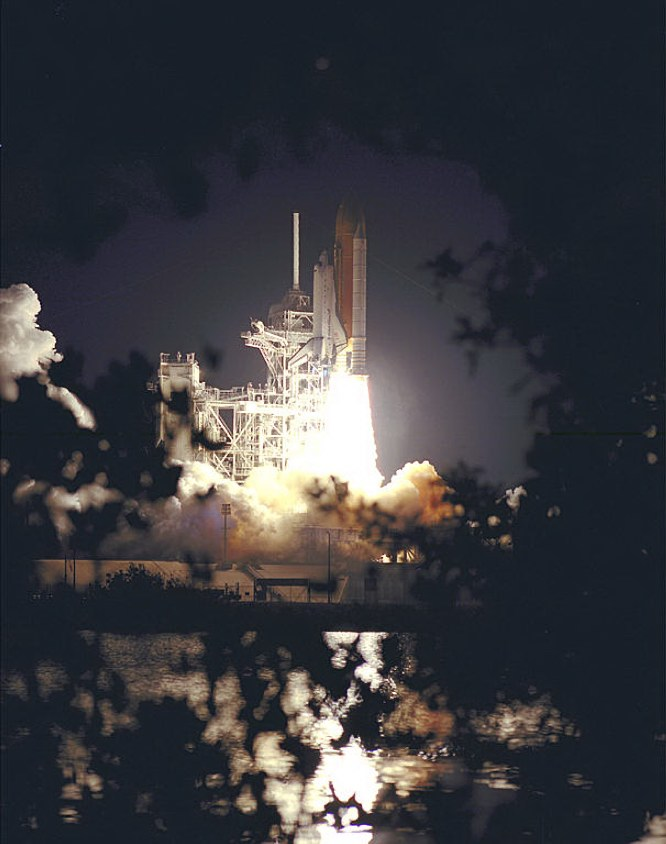STS-101 Space Shuttle Atlantis has liftoff

The crew repaired and installed a myriad of electrical and life-support components, both inside and out, and boosted the Station to a safe orbit. Webers two primary responsibilities were flying the 60-foot robotic arm to maneuver a spacewalk crewmember along the Station surface, and directing the transfer of over three thousand pounds of equipment.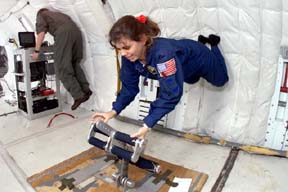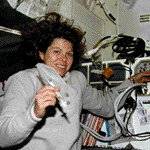STS-101 Atlantis landing at KSCSTS-101 crew greet onlookers after successful mission

The STS-101 mission was accomplished in 155 orbits of the Earth, after traveling 4.1 million miles in 236 hours and 9 minutes.

STS-70 Discovery (July 13-22, 1995), a mission which successfully delivered to orbit a critical NASA communications satellite, TDRS-G.STS-70 Discovery launch

Webers primary responsibility was checking the systems of the satellite and sending it into its 22-thousand-mile orbit above the equator.
She also performed biotechnology experiments, growing colon cancer tissues never before possible. She was the primary contingency spacewalk crewmember, and the medical officer.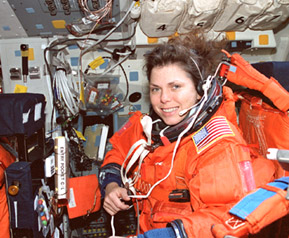The STS-70 mission was completed in 142 orbits of the Earth, after traveling 3.7 million miles in 214 hours and 20 minutes.

After NASA
Weber is currently (January 2003) Associate Vice President at the UT Southwestern Medical Center in Dallas, Texas.

Personal
Weber is married to Dr. Jerome Elkind, who is originally from Bayonne, New Jersey. She is an avid skydiver and golfer, and also enjoys scuba diving.<<

http://en.wikipedia.org/wiki/Mary_Ellen_Weber

____________________________

Assuming noon in Cleveland, Ohio (Lat41n30 - Lon81w42), 16:00 UT

Using RIYAL 3.1

Astrological Setting (Tropical - Placidus)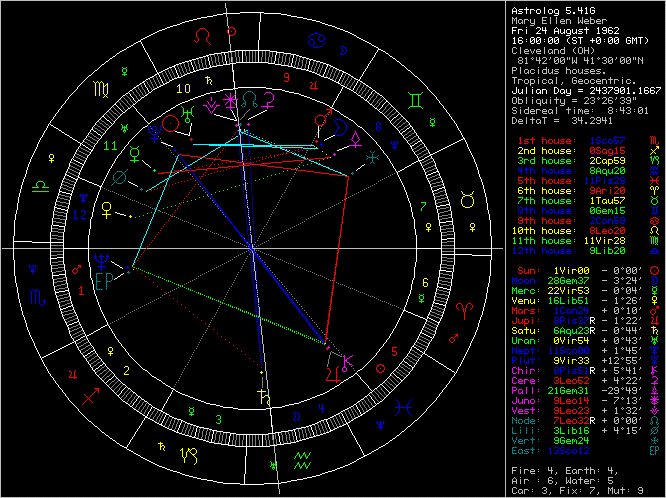RIYAL  Fri August 24 1962  UT 16h00m00s  Lat41n30  Lon81w42   SORT ALL

Planet     Longit.   Latit.   Declin.   Const.

RP120    =  0Sc05     13s10   23s46   Hya
FZ53     =  0Ar09 r   15n34   14n18   Peg
RR43     =  0Pi13 r   26s53   36s14   PsA
CE10     =  0Li29     20s50   19s14   Crt
Uranus   =  0Vi55      0n43   11n49   Leo
Sun      =  1Vi00      0n00   11n07   Leo
KF77     =  1Le11      4n04   23n52   Cnc
OM67     =  1Cp13 r   19s31   42s57   CrA
Mars     =  1Ca24      0n10   23n37   Gem
Ceto     =  1Ta47 r   14s38    1s40   Cet
(Ascend)   =  1Sc56      0n00   12s09   Vir
VR130    =  2Sa04      3n08   17s30   Sco
EL61     =  2Vi09     15n02   24n41   LMi
Nessus   =  2Ge12      7n50   28n16   Tau
DH5      =  3Pi05 r    1n29    8s59   Aqr
WL7      =  3Sa28      9s52   30s31   Lup
GM137    =  3Aq31 r   10s33   29s36   Mic
TL66     =  4Pi17 r   22n06   10n40   Peg
PN34     =  4Vi34      9s42    0n48   Sex
Hylonome =  5Ge11      3s46   17n28   Tau
Echeclus =  5Vi12      1s14    8n28   Leo
Teharonhi=  5Cp31 r    1s12   24s32   Sgr
FY9      =  5Le35     21n22   39n28   Lyn
LE31     =  5Ca48      9s20   13n59   Gem
Saturn   =  6Aq23 r    0s44   19s23   Cap
AZ84     =  6Ge46      1n26   22n51   Tau
AW197    =  6Ca56      9n06   32n20   Aur
XA255    =  7Ta45 r   12s01    2n42   Cet
OX3      =  8Sa03      0s57   22s35   Oph
(Midheav)  =  8Le20      0n00   18n11   Cnc
Jupiter  =  8Pi38 r    1s22    9s36   Aqr
Node     =  8Le50      0n00   18n03   Cnc
Chiron   =  8Pi51 r    5n41    2s59   Aqr
PB112    =  9Cp02 r   15s11   38s17   CrA
UX25     =  9Pi10 r   14n20    5n10   Peg
OO67     =  9Sa11 r   19n09    2s53   Oph
Vertex   =  9Ge24
Crantor  =  9Ta27 r   12s46    2n30   Cet
Pluto    =  9Vi33     12n55   19n55   Leo
Deucalion= 10Vi01      0n20    8n08   Leo
Huya     = 10Le06      9s42    8n21   Cnc
OP32     = 10Sa36     25n49    3n33   Oph
Eris     = 10Ar37 r   22s33   16s30   Cet
Okyrhoe  = 10Sc42     12n24    3s14   Lib
Apogee   = 10Vi58      2n49   10n03   Leo
Neptune  = 11Sc00      1n45   13s28   Lib
SQ73     = 11Vi08      9n39   16n17   Leo
DA62     = 11Sc16     25s21   39s03   Cen
TX300    = 11Aq20 r    6s00   23s09   Cap
Orcus    = 11Ca42      4s37   18n20   Gem
SA278    = 12Pi05 r    2n20    4s52   Aqr
CZ118    = 12Le14     15n39   32n05   Cnc
GB32     = 12Le18      9s54    7n36   Cnc
Chaos    = 12Ar20 r    7s36    2s08   Cet
MS4      = 12Sc32      2n34   13s09   Lib
Elatus   = 12Le37      3n36   20n29   Cnc
CF119    = 12Ge57     15n31   37n44   Per
CO104    = 13Ar41 r    1n03    6n22   Psc
QD112    = 14Ca14     13n51   36n26   Aur
Pholus   = 14Aq26 r    7s31   23s41   Cap
Logos    = 14Ca29      1s22   21n18   Gem
QB243    = 15Le14      1n42   17n54   Cnc
CY118    = 15Ca23     21s49    0n52   Mon
VS2      = 15Pi40 r   10n29    4n01   Psc
VU2      = 15Aq46 r   15n17    1s29   Aqr
Thereus  = 16Sa04     18n07    4s41   Oph
96PW     = 16Vi09     12n01   16n30   Leo
Chariklo = 16Aq20 r    7n43    8s34   Aqr
HB57     = 16Le37     13s24    3n03   Hya
QB1      = 16Aq46 r    1s29   17s14   Cap
Venus    = 16Li51      1s26    7s57   Vir
SB60     = 17Cp04 r    3n50   18s33   Sgr
VQ94     = 17Ar34 r   41s18   31s01   For
Amycus   = 18Ta00 r   13n26   30n03   Ari
XR190    = 18Ta17 r   23n51   39n59   And
TD10     = 18Sc25      4n12   13s16   Lib
XZ255    = 18Sc56      1n06   16s24   Lib
TO66     = 19Aq36 r   16s53   30s53   PsA
Quaoar   = 20Li18      1n46    6s18   Vir
GV9      = 20Le49     20s20    4s46   Hya
Varuna   = 21Ta06 r   12s49    5n39   Tau
BL41     = 21Aq12 r    9n36    5s20   Aqr
PA44     = 21Ge13      2s20   20n50   Tau
GZ32     = 21Ge16      6s58   16n12   Tau
CO1      = 22Ar09 r   17s11    7s20   Cet
Typhon   = 22Ar18 r    1n13    9n49   Psc
Mercury  = 22Vi53      0s04    2n46   Leo
BU48     = 22Pi54 r    9s36   11s37   Aqr
RN43     = 23Sa07 r   19n03    4s14   Oph
MW12     = 23Li15      7n48    1s47   Vir
GQ21     = 23Le23     10s09    4n08   Hya
CR105    = 23Ge42     16s28    6n50   Ori
Cyllarus = 23Sc53      1s01   19s43   Lib
PJ30     = 24Li19      5s28   14s30   Vir
WN188    = 24Aq29 r   11s08   23s50   Aqr
TY364    = 24Aq33 r    2s14   15s27   Cap
RZ214    = 24Cp46 r   20n44    0s45   Aql
Bienor   = 24Le55      4n30   17n27   Leo
FP185    = 24Ca57     27s53    6s20   Mon
QF6      = 25Sa01 r   20n32    2s50   Oph
Asbolus  = 25Ca28     16n17   37n01   Lyn
RM43     = 25Aq33 r   26s17   37s27   Gru
Ixion    = 25Li43     13n42    2n51   Vir
FZ173    = 26Ca03     11n30   32n13   Gem
Pelion   = 26Le40      3n02   15n28   Leo
CC22     = 27Ge13      5s07   18n18   Ori
TC302    = 28Pi00 r   17s14   16s34   Cet
UJ438    = 28Sa13 r    0n31   22s54   Sgr
YQ179    = 28Ta15     16s46    3n24   Tau
RG33     = 28Le25     27n57   37n53   LMi
(Moon)     = 28Ge37      3s24   20n02   Ori
RD215    = 28Cp44 r   26n26    5n31   Aql
Sedna    = 28Ar44 r   10s51    0n53   Cet
UR163    = 28Aq50 r    0n21   11s33   Aqr
RZ215    = 28Sc53     25s05   44s13   Lup
RL43     = 29Sc04     12n03    8s10   Lib
KX14     = 29Vi09      0s23    0n00   Vir
XX143    = 29Li10      6n14    5s21   Vir
Pylenor  = 29Le39      1n05   12n36   Leo
__________________________

Focused Minor Planets

EL61     =  2 Vi 09   (Needing physical/psychological restraints, in order to
disclose powerful energy)
Sun       =  1 Vi 00
Uranus =  0 Vi 55
RR43     =  0 Pi 13 r

Mars      =  1 Ca 24       Sextile

Venus   = 16 Li 51        Semisquare

OM67    =   1 Cp 13 r    Trine
Ceto      =   1 Ta 47 r

Nessus  =   2 Ge 12      Square
____________________

CR105   =   23 Ge 42
RN43     =   23 Sa 07 r

Mercury =  22 Vi 53      Square
BU48      =   22 Pi 54 r

TY364    =   24 Aq 33 r  Trine
RM43     =   25 Aq 33 r
MW12    =   23 Li 15

Node      =     8 Le 50      Semisquare

Typhon   =   22 Ar 18 r     Sextile
GQ21     =   23 Le 23
Bienor    =   24 Le 55

Cyllarus  =   23 Sc 53      Quincunx
____________________

Varuna   =  21 Ta 06 r

Mercury =  22 Vi 53       Trine

AW197   =     6 Ca 56     Semisquare
____________________

SB60     =   17 Cp 04 r

Venus   =   16 Li 51        Square

QB1       =   16 Aq 46 r    Semisextile
Chariklo =  16 Aq 20 r
Thereus  =  16 Sa 04

HB57     =   16 Le 37       Quincunx
____________________

UX25     =  9 Pi 10 r
Jupiter  =  8 Pi 38 r
Chiron    =  8 Pi 51 r
Pluto      =  9 Vi 33
Deucalion = 10 Vi 01

OO67     =   9 Sa 11 r     Square

Node      =   8 Le 50       Quincunx

Crantor  =   9 Ta 27 r      Sextile
PB112   =   9 Cp 02 r
____________________

FY9       =     5 Le 35
Saturn  =     6 Aq 23 r

TL66     =     4 Pi 17 r    Quincunx
Teharonhi =  5 Cp 31 r

Echeclus  =  5 Vi 12      Semisextile

Hylonome =  5 Ge 11    Sextile
____________________

AW197    =   6 Ca 56

Saturn    =   6 Aq 23 r    Quincunx

Jupiter   =   8 Pi 38 r      Trine

Varuna   = 21 Ta 06 r     Semisquare
____________________

Orcus     =  11 Ca 42

Neptune = 11 Sc 00      Trine
Okyrhoe   = 10 Sc 42
SA278     = 12 Pi 05 r

Eris       =    10 Ar 37 r     Square
Chaos  =    12 Ar 20 r

TX300   =    11 Aq 20 r    Quincunx
OP32    =    10 Sa 36
_______________________________
_______________________________

RIYAL  Fri August 24 1962  UT 16h00m00s  Lat41n30  Lon81w42   SORT ALL

Planet      Longit.

PJ30     =  0Li06
WN188    =  0Aq16 r
TY364    =  0Aq20 r
RZ214    =  0Cp33 r
Bienor   =  0Le42
FP185    =  0Ca44
QF6      =  0Sa48 r
Asbolus  =  1Ca15
RM43     =  1Aq20 r
Ixion    =  1Li30
FZ173    =  1Ca50
Pelion   =  2Le27
CC22     =  3Ge00
TC302    =  3Pi47 r
UJ438    =  4Sa00 r
YQ179    =  4Ta02
RG33     =  4Le12
(Moon)     =  4Ge24
RD215    =  4Cp31 r
Sedna    =  4Ar31 r
UR163    =  4Aq37 r
RZ215    =  4Sc40
RL43     =  4Sc51
KX14     =  4Vi56
XX143    =  4Li57
Pylenor  =  5Le27
RP120    =  5Li52
FZ53     =  5Pi56 r
RR43     =  6Aq00 r
CE10     =  6Vi16
Uranus  =  6Le42
Sun      =  6Le47
KF77     =  6Ca58
OM67     =  7Sa00 r
Mars     =  7Ge11
Ceto     =  7Ar34 r
(Ascend)   =  7Li43
VR130    =  7Sc51
EL61     =  7Le56
Nessus   =  7Ta59
DH5      =  8Aq52 r
WL7      =  9Sc15
GM137    =  9Cp18 r
TL66     = 10Aq04 r
PN34     = 10Le21
Hylonome = 10Ta58
Echeclus = 10Le59
Teharonhi= 11Sa18 r
FY9      = 11Ca22
LE31     = 11Ge35
Saturn   = 12Cp10 r
AZ84     = 12Ta33
AW197    = 12Ge43
XA255    = 13Ar32 r
OX3      = 13Sc50
(Midheav)  = 14Ca07
Jupiter  = 14Aq25 r
Node     = 14Ca38
Chiron   = 14Aq38 r
PB112    = 14Sa50 r
UX25     = 14Aq57 r
OO67     = 14Sc58 r
Vertex   = 15Ta11
Crantor  = 15Ar14 r
Pluto    = 15Le20
Deucalion= 15Le48
Huya     = 15Ca53
OP32     = 16Sc23
Eris     = 16Pi24 r
Okyrhoe  = 16Li29
Apogee   = 16Le45
Neptune  = 16Li47
SQ73     = 16Le55
DA62     = 17Li03
TX300    = 17Cp07 r
Orcus    = 17Ge29
SA278    = 17Aq52 r
CZ118    = 18Ca01
GB32     = 18Ca05
Chaos    = 18Pi07 r
MS4      = 18Li19
Elatus   = 18Ca24
CF119    = 18Ta44
CO104    = 19Pi28 r
QD112    = 20Ge01
Pholus   = 20Cp13 r
Logos    = 20Ge16
QB243    = 21Ca01
CY118    = 21Ge11
VS2      = 21Aq27 r
VU2      = 21Cp33 r
Thereus  = 21Sc51
96PW     = 21Le56
Chariklo = 22Cp07 r
HB57     = 22Ca24
QB1      = 22Cp33 r
Venus    = 22Vi38
SB60     = 22Sa51 r
VQ94     = 23Pi21 r
Amycus   = 23Ar47 r
XR190    = 24Ar04 r
TD10     = 24Li12
XZ255    = 24Li44
TO66     = 25Cp23 r
Quaoar   = 26Vi05
GV9      = 26Ca37
Varuna   = 26Ar53 r
BL41     = 26Cp59 r
PA44     = 27Ta00
GZ32     = 27Ta04
CO1      = 27Pi56 r
Typhon   = 28Pi05 r
Mercury  = 28Le40
BU48     = 28Aq41 r
RN43     = 28Sc54 r
MW12     = 29Vi02
GQ21     = 29Ca10
CR105    = 29Ta30
Cyllarus = 29Li40
___________________

Focused Minor Planets

EL61     =   7 Le 56   (Needing physical/psychological restraints, in order to
disclose powerful energy)
Sun       =   6 Le 47
Uranus =   6 Le 42
RR43     =   6 Aq 00 r

Mars     =    7 Ge 11      Sextile

Venus  =  22 Vi 38       Semisquare

OM67   =    7 Sa 00 r    Trine
Ceto     =    7 Ar 34 r

Nessus =    7 Ta 59       Square
____________________

CR105   =   29 Ta 30
RN43     =   28 Sc 54 r

Mercury =  28 Le 40      Square
BU48      =  28 Aq 41 r

TY364    =    0 Aq 20 r    Trine
RM43     =    1 Aq 20 r
MW12    =  29 Vi 02

Node      =  14 Ca 38      Semisquare

Typhon   =  28 Pi 05 r      Sextile
GQ21     =  29 Ca 10
Bienor    =    0 Le 42

Cyllarus  = 29 Li 40         Quincunx
____________________

Varuna   =  26 Ar 53 r

Mercury =  28 Le 40      Trine

AW197    = 12 Ge 43     Semisquare
____________________

SB60     =  22 Sa 51 r

Venus   =  22 Vi 38        Square

QB1       =  22 Cp 33 r    Semisextile
Chariklo =  22 Cp 07 r
Thereus  =  21 Sc 51

HB57      =  22 Ca 24     Quincunx
____________________

UX25     =  14 Aq 57 r
Jupiter  =  14 Aq 25 r
Chiron   =  14 Aq 38 r
Pluto    =  15 Le 20
Deucalion = 15 Le 48

OO67     = 14 Sc 58 r     Square

Node     =  14 Ca 38       Quincunx

Crantor  = 15 Ar 14 r      Sextile
PB112   = 14 Sa 50 r
____________________

FY9         =   11 Ca 22
Saturn   =   12 Cp 10 r

TL66      =    10 Aq 04 r   Quincunx
Teharonhi =  11 Sa 18 r

Echeclus  =  10 Le 59     Semisextile

Hylonome = 10 Ta 58      Sextile
____________________

AW197    =   12 Ge 43

Saturn    =   12 Cp 10 r   Quincunx

Jupiter    =   14 Aq 25 r   Trine

Varuna    =   26 Ar 53 r    Semisquare
____________________

Orcus     =   17 Ge 29

Neptune =  16 Li 47        Trine
Okyrhoe  =   16 Li 29
SA278    =   17 Aq 52 r

Eris         =   16 Pi 24 r     Square
Chaos    =   18 Pi 07 r

TX300    =    17 Cp 07 r   Quincunx
OP32     =    16 Sc 23
_______________________________

On the basis of this case and other data, it seems possible to try an integration with Roy McKinnon's "egg - shell" hypothesis about EL61, that may be the following key phrase:
"Needing physical/psychological restraints, in order to disclose powerful energy".
Physical/psychological restraints = a sort of shell.
A spacecraft is a sort of shell, inducing both physical, psychological restraint.
----------------------------

Perhaps, other potential keywords for 2003 EL61 are in this site:

http://maryellenweber.com/index.html
________________________________________________

Posted to Centaurs (YahooGroups) on July 30, 2008

_______________________________________
_______________________________________